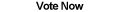Home Articles Components Code Snippets Links# C# Decimal To Fraction

## Double to Fraction

Sometimes it is useful to represent decimal numbers as fractions in C#. Using a simple algorithm, we can approximate decimals values to a fraction representation.

## Direct Algorithm

The direct way to turn a decimal number into a fraction is to write the number as a fraction of 10. What does that mean? Take 0.4 for example. It is the same as saying 4/10, and by reducing, we get the fraction 2/5. Have about 0.125? That is 125/1000 which reduces to 1/8.

What about 0.753543? Technically that is the same as 753543/1000000, which we can also reduce. How about 0.7535234236233? Starting with the direct fraction gets kind of ridiculous at this point.

But take that 0.7535234236233. we can round the number to 0.75, which is 3/4. So 3/4 is not the exact fraction, but it is a close approximation.

Thus our algorithm can be more efficient by simply focusing on getting a fraction that is within a certain range from the actual value. An approximation.

## Approximation Algorithm

One algorithm I really liked can be found on this math website. (There are two algorithms described, I am talking about the first one). This algorithm is fairly simple to execute. Below is my attempt to explain it:

For a given decimal number, 3.43, separate the whole number (3) from the decimal portion (0.43). Using the decimal portion, we calculate a new value. Let's call it value "x". The resulting fraction will be 1 / (3 + x). What is x? "x" is the same algorithm applied on the result of 1/0.43, which in this case is 2.325...etc.

If you did not understand that at all, check out the link above. They do a much better job of explaining it.

As you can tell this is a perfect place to implement a recursive algorithm since the value "x" is the result of the same function. But there are two important things to we must address before implementing it.

First is the most important rule of a recursive function: when do we stop? As the math page describes, a good place to stop is when the (1/decimal portion) value is really small. So small that it can be considered 0. Then the rest of the call can finish adding the accumulated values until we get the final (1 / whole number + x) value.

However here is were we have another challege. "x" is a fraction (since the result of our function is turning a decimal into a fraction). How do we add x to that value without ending up with a decimal again? More importantly, once we add it, we are going to end up with something like 1 / (8 / 3). Using simple fraction math we know that the answer is 3 / 8, but how can we implement this?

This is where C#'s object-oriented design comes in. We can simply use a fraction C# structure to encapsulate all the fraction math (including adding, dividing, reducing, simplifying) and let the algorithm just worry about executing the logic.

## Implementation

There are still a couple things to consider before implementing the C# code. We agreed that recursion would stop when (1 / decimal value) got too small. But what is too small? In this case we are going to use Double.Epsilon by default and provide an overload for the user to specify their own bounds.

The problem now is that values will never be smaller than Epsilon unless it is actually zero (or is at least computed to be zero). We can solve this by providing a maximum number of recursive calls. With each call, the value is decreased, once it reaches 0, the recursive call will return 0 no matter what.

With those two things, we can now implement the algorithm. Remember that we need a structure to represent fractions in C#. The actual code to convert a double to a fraction is straightforward but a bit a long, so it is included as a download below.

Back to C# Article List